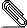```Hi,

On Fri, Sep 21, 2007 at 12:42:36AM -0700, mpb wrote:
> always canonicalize paths.  It may be possible to create a simpler
> demonstration of the bug, but I could not trivially do so.
[...]
> path=/tmp/c0/b3/b4/a5/a6
[...]
```

\$ strace -e lstat64 stat -c '' /tmp/c0/b3/b4/a5/a6
lstat64("/tmp/c0/b3/b4/a5/a6", {st_mode=S_IFREG|0644, st_size=9, ...}) = 0
lstat64("/tmp", {st_mode=S_IFDIR|S_ISVTX|0777, st_size=4096, ...}) = 0
lstat64("/tmp/c0", {st_mode=S_IFDIR|0755, st_size=4096, ...}) = 0
lstat64("/tmp/c0/b3", {st_mode=S_IFLNK|0777, st_size=13, ...}) = 0
lstat64("/tmp", {st_mode=S_IFDIR|S_ISVTX|0777, st_size=4096, ...}) = 0
lstat64("/tmp/a0", {st_mode=S_IFDIR|0755, st_size=4096, ...}) = 0
lstat64("/tmp/a0/a2", {st_mode=S_IFLNK|0777, st_size=5, ...}) = 0
lstat64("/tmp/a0/a1", {st_mode=S_IFDIR|0755, st_size=4096, ...}) = 0
lstat64("/tmp/a0/a1/a2", {st_mode=S_IFDIR|0755, st_size=4096, ...}) = 0
lstat64("/tmp/a0/a1/a2/b3", {st_mode=S_IFDIR|0755, st_size=4096, ...}) = 0
lstat64("/tmp/a0/a1/a2/b3/b4", {st_mode=S_IFDIR|0755, st_size=4096, ...}) = 0
lstat64("/tmp/a0/a1/a2/b3/b4/a5", {st_mode=S_IFLNK|0777, st_size=19, ...}) = 0
lstat64("/tmp", {st_mode=S_IFDIR|S_ISVTX|0777, st_size=4096, ...}) = 0
lstat64("/tmp/a0", {st_mode=S_IFDIR|0755, st_size=4096, ...}) = 0
lstat64("/tmp/a0/a2", {st_mode=S_IFLNK|0777, st_size=5, ...}) = 0

That is, /tmp/a0/a2 is checked twice, and cycle_check() aborts the
traversal.

--
ldv
```pgpIjTi4ntcB6.pgp
Description: PGP signature

```_______________________________________________
Bug-coreutils mailing list
Bug-coreutils@gnu.org
http://lists.gnu.org/mailman/listinfo/bug-coreutils
```